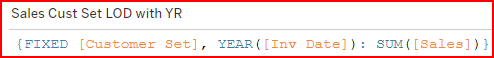# Table Calculations - the next level

### Table calculations

1. Table calculations compute using the data on the screen rather than the data in the dataset.

2. The most commonly used table calculations are: Difference, % Difference, Rank, % Total, Running Total

3. You can modify the Table calculation to either look at the entire table of data on the screen, or only scan data within a specific pane at a time

4. Table calculations can go from basic to complex very quickly. The help topics listed above are for those who want to go beyond the common uses.

### Using Table Calculations for Totals and Sub TotalsIn cases where the Dimension field used in the calculation is not on the worksheet, then an LOD calculation can work in place of a Table Calculation.

#### Activity: Create Table Calculations to show % of set, and % of grand total.

Convert the Sum (Sales) Measure Value to a Quick Table Calculation % of Grand total.

Save the Table Calculation to the Data Pane and rename it `% Grand Total`. Then replace the original Table Calculation. Add the Sum (Sales) again.

Convert the Sum (Sales) Measure Value to a Quick Table Calculation % of Set Total
Then edit the Table Calculation to compute using Pane (down).

Save the Table Calculation to the Data Pane and rename it % Set Total. Then replace the original Table Calculation. Finally, add the Sum (Sales) again.#### Using LODs to show the Set subtotal as a percent of grand total `Set % GT`.

An alternative to using a Table Calculation to calculate the % Grand Total per Set is to use an LOD calculation. The example below is for reference.

1. Duplicate the worksheet you created for the Set Actions

2. Create an LOD calculated field to show the Sum (Sales) per Customer Set output (IN/OUT)LOD (including YEAR) to show sum of sales per Customer Set
3. Create another LOD calculation to show the grand total of sales per year.